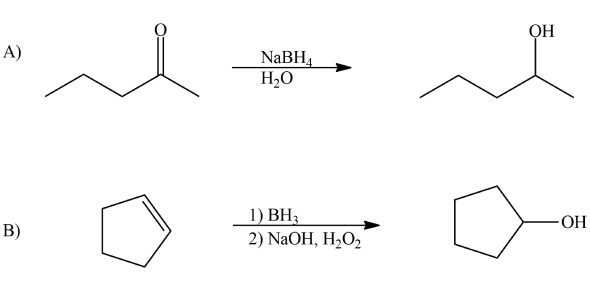# Oxidation And Reduction Reactions Quiz! Trivia

19 Questions | Attempts: 4434SettingsBelow is an Oxidation and reduction reactions quiz trivia. Oxidation is the process where electrons are lost while reduction is the transfer of electrons between substances. Oxygen is either lost or gained in the reactions. In this quiz you will test out what you know about different compounds and substances and what happens when they undergo this process. Do give it a shot and see how well you do!

• 1.
The terms Oxidation and Reduction both have direct polar opposite definitions.  what is each term defined as?
• A.

Oxidation- the loss of electrons by atom or ion/ the gain of oxygen, Reduction- the loss of oxygen/ the gain of said electrons.

• B.

Oxidation- the gain of electrons by an atom or ion/ loss of oxygen, Reduction- the gain of oxygen/ the loss of electrons by atom or ion.

• C.

Oxidation- something having to do with oxide, i don't care -__- Reduction- something's being reduced, but i don't know what.

• 2.
Can oxidation and reduction occur without one another?
• A.

They are interdependent

• B.

They are not interdependent.

• 3.
What, in simplified terms, does Redox mean?
• A.

Its a combination of Reduction and Oxidation, when one occurs, the other does not.

• B.

It is not a combination of reduction and oxidation , when one occurs, the other must occur as well.

• C.

Its a combo of both REDuction and OXidation. one reaction cannot occur without the other.

• 4.
What does LEO say GER stand for?
• A.

LEO- Loss of Electrons is Oxygen, GER- Gain of Electrons is Red

• B.

LEO- Loss of Elections is Oxidation, GER- Gain of Electrons is Reduction

• C.

LEO- Loss of Electrons is Oxidation, GER- Gain of Electrons is Reduction

• 5.
Credit given only to those who show all work!! what are the oxidation numbers of the atoms in HNO3?
• A.

H: +1, N: +5 , O: -2

• B.

H: 1, N: 5, O: 2

• C.

H: +1, N: -5, O: +2

• 6.
The sum of the oxidation numbers in all compounds is equal to 1.
• A.

True

• B.

False

• 7.
The sum of the oxidation numbers in all compounds is equal to 0.
• A.

True

• B.

False

• 8.
The sum of the oxidation numbers in the polyatomic ions must be equal to the charge on the ion.
• A.

True

• B.

False

• 9.
In which substance does phosphorus have a +3 oxidation state
• A.

P4O10

• B.

PCl5

• C.

Ca3(PO4)2

• D.

KH2PO3

• 10.
In order for an equation to be a REDOX equation, you must recognize it as a..?
• 11.
Which equation represents an oxidation-reduction reaction?
• A.

HCl + KOH yields KCl + H2O

• B.

4HCl + MnO2 yields MnCl2 + 2H2O +Cl2

• C.

2HCl +CaCO3 yields CaCl2 + H2O +CO2

• D.

2Hcl + FeS yields FeCl2 +H2S

• 12.
When a Substance is oxidized, it
• A.

Loses protons

• B.

Gains protons

• C.

Acts like a reducing agent

• D.

Acts as an oxidizing agent

• E.

Its gets oxyclean. xD

• 13.
What do you call the substance being reduced?
• 14.
What do you call the substance being oxidized?
• 15.
Consider the Following Equation. 2Fe3+ + Sn2+ yields 2Fe2++Sn4+ who is the oxidizing agent?
• A.

Fe3+

• B.

Sn2+

• C.

Fe2+

• D.

Sn4+

• 16.
If the number changes from high oxidation to low, then it has been oxidized.
• A.

True

• B.

False

• 17.
In the Equation  MnO2 + 4HCl yields MnCl2 + Cl2 + 2H2O what is the Reducing Agent and is the Oxidizing Agent?
• 18.
Oxidation-Reduction Reactions occur because of the competition between particles for
• A.

Neutrons

• B.

Elections

• C.

Positrons

• D.

Electrons

• 19.
What must ALWAYS occur in REDOX reactions?

## Related TopicsBack to top
×

Wait!
Here's an interesting quiz for you.